# Rational polynomial representation of ribonucleotide reductase activity

## Abstract

### Background

Recent data suggest that ribonucleotide reductase (RNR) exists not only as a heterodimer R12R22 of R12 and R22 homodimers, but also as tetramers R14R24 and hexamers R16R26. Recent data also suggest that ATP binds the R1 subunit at a previously undescribed hexamerization site, in addition to its binding to previously described dimerization and tetramerization sites. Thus, the current view is that R1 has four NDP substrate binding possibilities, four dimerization site binding possibilities (dATP, ATP, dGTP, or dTTP), two tetramerization site binding possibilities (dATP or ATP), and one hexamerization site binding possibility (ATP), in addition to possibilities of unbound site states. This large number of internal R1 states implies an even larger number of quaternary states. A mathematical model of RNR activity which explicitly represents the states of R1 currently exists, but it is complicated in several ways: (1) it includes up to six-fold nested sums; (2) it uses different mathematical structures under different substrate-modulator conditions; and (3) it requires root solutions of high order polynomials to determine R1 proportions in mono-, di-, tetra- and hexamer states and thus RNR activity as a function of modulator and total R1 concentrations.

### Results

We present four (one for each NDP) rational polynomial models of RNR activity as a function of substrate and reaction rate modifier concentrations. The new models avoid the complications of the earlier model without compromising curve fits to recent data.

### Conclusion

Compared to the earlier model of recent data, the new rational polynomial models are simpler, adequately fitting, and likely better suited for biochemical network simulations.

## Background

Ribonucleotide reductase (RNR) is a key component of de novo deoxynucleotide (dNTP) metabolism and an important target of cancer therapies . This enzyme, which reduces ribonucleoside diphosphates into corresponding deoxyribonucleoside diphosphates, is exquisitely controlled to properly balance dNTP fluxes in the face of changing scheduled (S phase) and unscheduled (DNA damage/repair) dNTP synthesis demands .

Recent data  suggest that ribonucleotide reductase (RNR) exists not only as a heterodimer R12R22 of R12 and R22 homodimers , but also as a R14R24 tetramer and as a R16R26 hexamer, where hexamer formation is driven by ATP binding to a previously undescribed hexamerization site. Thus, in addition to its four substrate binding possibilities in ADP, GDP, CDP, or UDP, and four dimerization/specificity site binding possibilities in dATP, ATP, dGTP, or dTTP, the current view (Figure 1) is that R1 has two tetramerization/inhibitory site binding possibilities in dATP or ATP, and one hexamerization/activation site binding possibility in ATP, in addition to possibilities of unbound site states. The resulting large number of possible R1 states implies an even larger number of quaternary states, and this leads to a complicated mathematical model of RNR activity . This model, although useful for explaining RNR activity data, is not useful for biochemical network simulations because: a) it is unwieldy (including up to six-fold nested sums), b) it uses different mathematical structures under different substrate-modulator conditions, and c) it requires root solutions of high order polynomials to determine R1 proportions in mono-, di-, tetra- and hexamer quaternary states, and thus RNR activity, as a function of modulator and total R1 concentrations. Simpler mathematical reaction rate models of RNR are needed if deoxynucleotide metabolism  is to be represented using Systems Biology Markup Language (SBML) , a standard which requires single algebraic expression reaction rate laws in some applications [11, 12]. Based on recent data from Cooperman's group , such expressions are provided here for RNR.

## Results

Reaction activities are viewed here as weighted sums of enzyme state specific activities multiplied by probabilities of enzymes being in specific states. For example, a Michaelis-Menten reaction rate law is viewed aswhere the probability that the enzyme is in a loaded/reactive state (with activity kcat) is P(EA) and the probability that the enzyme is in an empty/unreactive state (with no activity) is P(E).

The RNR models presented here are based on the following four enzyme state probability assumptions:

1. The probability that a particular R1 subunit is bound to NDP is assumed to be2. The probability that L {ATP, dATP, dTTP, dGTP} is also bound to the dimerization/specificity site, conditional on NDP binding, is assumed to be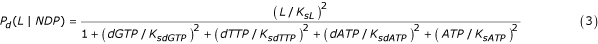where, if L = dTTP and NDP = GDP for example,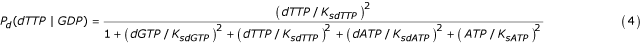is the probability that dTTP is bound to the dimerization/specificity site given that GDP is bound to the substrate site; the probability of an empty dimerization/specificity site is thus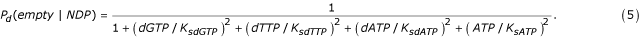3. The probability that the tetramerization site is either empty, occupied by ATP, or occupied by dATP, is assumed to be, respectively,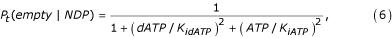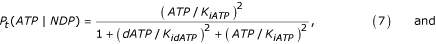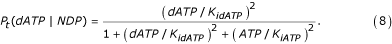4. Finally, the probability that the hexamerization site is occupied by ATP is assumed to beThe subscripts a (activation), i (inactivation), and s (specificity) on the binding constants correspond to h (hexamerization), t (tetramerization), and d (dimerization) subscripts on P, respectively. That parameter values differ depending upon which NDP substrate is bound to the active site (see Table 1) is indicated by the conditional probabilities.

Previous work  has shown that the dimer and hexamer states are active, that the tetramer state is slightly active for ADP and GDP and is otherwise inactive, that dimer state activity for CDP and UDP exists when ATP or dATP is bound to the dimerization/specificity site, and that an empty dimerization/specificity site still permits the formation of some dimer with CDP reductase activity, see Table 5 of . Thus, based on the enzyme state probabilities given above, for kcat implicitly defined throughwe propose the following expressions:GDP reductionCDP reductionUDP reduction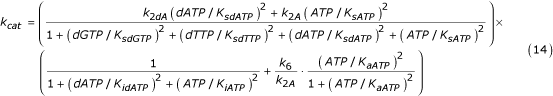In these equations, for ADP and GDP, the first factor is the probability that the dimer site is occupied, and for CDP and UDP, the first factor is the expectation of kcat conditional on R1 being in a dimer state (i.e. having an empty tetramerization site). In the ADP and GDP models, the second factor is the conditional expectation of kcat given that the dimerization site is occupied: the first term of this second factor has in its numerator the statement that kcat = k2 if the tetramerization site is empty, or kcat = k4 if it is occupied by either dATP or ATP, and the second term states that kcat = k6 if the hexamerization site is occupied by ATP. For the CDP and UDP models, the first term of the second factor is the probability of an empty tetramerization site (the event that the corresponding first factor was conditioned on), and the second term states that if the ATP concentration is high enough that the hexamerization/second term dominates the tetramerization/first term whilst the first factor approaches k2A, k6 is the overall kcat. This rationale served as our model selection guide. Importantly, the models fitted recent data  very well, see Figure 2 and Table 1.

## Discussion

In general, when an integrated system is engineered from component subsystems, the behavior of the overall system depends on component input-output specifications more so than the details of component implementations. By analogy, when enzymological data are applied to biochemical network modeling, rather than the elucidation of reaction mechanisms, it can be expected that the reaction surfaces themselves (i.e. the enzyme's input-output characteristics) determine network behavior more so than the details of how such surfaces are represented. Thus, for applications to systems biology, large confidence intervals (CI) in the model parameter estimates of Table 1 (not shown) are not a problem because only goodness-of-fit (Fig. 2) really matters; this claim assumes an operating range within the data range, since similarly fitting models often veer apart when used in extrapolations. If reaction mechanism inferences were instead being sought, the large CI in the model parameter estimates would have been a problem, e.g. the squared terms in the model suggest cooperative binding, but this choice provides only slightly better curve fits compared to linear terms (not shown), so cooperative binding cannot be inferred from this model.

In the RNR model presented here, the proportion of R1 units existing in monomer, dimer, tetramer, or hexamer states, and thus the RNR activity per unit enzyme, depends on site binding occupancies but does not depend on the total R1 concentration. In the more complicated previous model , higher total R1 concentrations favor higher order quaternary states. The degree to which this is so is illustrated by plots of predicted GDP reductase activity as a function of ATP concentration at various R1 concentrations (Figure 3). Consistent with the formation of higher order quaternary R1 states, these plots contract to the left as the total R1 concentrations increase from 1 μM to 100 μM. In future work, the model given here will be altered to capture such trends without losing its simplicity; the total R1 concentration will enter such a model not only as a linear modulator of the reaction surface amplitude (i.e. E0 in Eq. 10), but also as a modifier of reaction surface shape parameters, e.g. KaATP will be replaced by a decreasing function of R1.

## Conclusion

We identified a rational polynomial model of RNR activity that has single algebraic expressions for each reductase reaction rate law. The expressions provide reasonably good fits (Fig. 2) to recent data . Compared to previous reaction rate expressions for this data , the new expressions are simpler and thus better suited for biochemical network simulations, particularly those constrained to use enzyme reaction rate laws defined as single algebraic expressions [11, 12].

## Methods

The parameter estimates shown in Table 1 were obtained through a trial-and-error iterative process of nonlinear least squares curve fitting under various, convergence enabling, parameter fixations (i.e. profile searches). In the end, the curve fits were those of Figure 2 with corresponding parameter estimates in Table 1; large 95% confidence intervals (not shown) allowed rounding of the parameter estimates to somewhat arbitrary choices. Non-linear least squares parameter estimations were performed using the optimization method of Nelder and Mead  and the statistical computing environment R . All parameters were estimated as ec to assure positive values. For additional details, R scripts are available with the data as supplementary material .

## Abbreviations

RNR:

= ribonucleotide reductase

dNTP:

= deoxynucleoside tripshospate

dNDP:

= deoxynucleoside dipshospate

a :

= activation

i :

= inactivation

s :

= specificity

h :

= hexamerization

t :

= tetramerization

d :

= dimerization

CI:

= confidence interval

SBML:

= Systems Biology Markup Language.

## References

1. Tsimberidou AM, Alvarado Y, Giles FJ: Evolving role of ribonucleoside reductase inhibitors in hematologic malignancies. Expert Rev Anticancer Ther. 2002, 2 (4): 437-448. 10.1586/14737140.2.4.437.

2. Kolberg M, Strand KR, Graff P, Andersson KK: Structure, function, and mechanism of ribonucleotide reductases. Biochim Biophys Acta. 2004, 1699 (1-2): 1-34.

3. Cooperman BS, Kashlan OB: A comprehensive model for the allosteric regulation of Class Ia ribonucleotide reductases. Adv Enzyme Regul. 2003, 43: 167-182. 10.1016/S0065-2571(02)00035-3.

4. Kashlan OB, Cooperman BS: Comprehensive model for allosteric regulation of mammalian ribonucleotide reductase: refinements and consequences. Biochemistry. 2003, 42 (6): 1696-1706. 10.1021/bi020634d.

5. Kashlan OB, Scott CP, Lear JD, Cooperman BS: A comprehensive model for the allosteric regulation of mammalian ribonucleotide reductase. Functional consequences of ATP- and dATP-induced oligomerization of the large subunit. Biochemistry. 2002, 41 (2): 462-474. 10.1021/bi011653a.

6. Scott CP, Kashlan OB, Lear JD, Cooperman BS: A quantitative model for allosteric control of purine reduction by murine ribonucleotide reductase. Biochemistry. 2001, 40 (6): 1651-1661. 10.1021/bi002335z.

7. Jackson RC: The Theoretical Foundations of Cancer Chemotherapy Introduced by Computer Models. 1992, New York , Academic Press, 447-

8. Hucka M, Finney A, Sauro HM, Bolouri H, Doyle JC, Kitano H, Arkin AP, Bornstein BJ, Bray D, Cornish-Bowden A, Cuellar AA, Dronov S, Gilles ED, Ginkel M, Gor V, Goryanin II, Hedley WJ, Hodgman TC, Hofmeyr JH, Hunter PJ, Juty NS, Kasberger JL, Kremling A, Kummer U, Le Novere N, Loew LM, Lucio D, Mendes P, Minch E, Mjolsness ED, Nakayama Y, Nelson MR, Nielsen PF, Sakurada T, Schaff JC, Shapiro BE, Shimizu TS, Spence HD, Stelling J, Takahashi K, Tomita M, Wagner J, Wang J: The systems biology markup language (SBML): a medium for representation and exchange of biochemical network models. Bioinformatics. 2003, 19 (4): 524-531. 10.1093/bioinformatics/btg015.

9. Finney A, Hucka M: Systems biology markup language: Level 2 and beyond. Biochem Soc Trans. 2003, 31 (Pt 6): 1472-1473.

10. Systems Biology Markup Language. [http://sbml.org]

11. Radivoyevitch T: A two-way interface between limited Systems Biology Markup Language and R. BMC Bioinformatics. 2004, 5 (1): 190-10.1186/1471-2105-5-190.

13. Nelder JA, Mead R: A simplex algorithm for function minimization. Computer Journal. 1965, 7: 308-313.

14. Ihaka R, Gentleman R: R:a language for data analysis and graphics. Journal of Computational and graphical statistics. 1996, 5: 299-314.

15. Radivoyevitch T, Kashlan OB, Cooperman BS: Rational Polynomial Representation of Ribonucleotide Reductase Activity. [http://epbi-radivot.cwru.edu/cooperman/]

## Acknowledgements

We thank Dr. Charles Scott for sharing his data. TR was supported by the Biostatistics Core Facility of the Comprehensive Cancer Center of Case Western Reserve University and University Hospitals of Cleveland (P30 CA43703), the American Cancer Society (IRG-91-022-09), the National Cancer Institute's Integrative Cancer Biology Program (P20 CA112963-01) and NIH grant 1K25 CA104791-01A1. BSC was supported by NIH grant CA 58567. OBK was a recipient of a postdoctoral fellowship award from the Pennsylvania-Delaware Affiliate of the AHA, and was supported by grants DK061296 and DK066883 from the NIH.

## Author information

Authors

### Authors' contributions

TR performed the curve fits to the data and explored various model choices.

OBK and BSC provided the original model, its simulations (Figure 3) and the data.

## Authors’ original submitted files for images

Below are the links to the authors’ original submitted files for images.

## Rights and permissions

Reprints and Permissions

Radivoyevitch, T., Kashlan, O.B. & Cooperman, B.S. Rational polynomial representation of ribonucleotide reductase activity. BMC Biochem 6, 8 (2005). https://doi.org/10.1186/1471-2091-6-8

• Accepted:

• Published:

• DOI: https://doi.org/10.1186/1471-2091-6-8

### Keywords

• Ribonucleotide Reductase
• System Biology Markup Language
• Dimerization Site
• Ribonucleotide Reductase Activity
• Quaternary State